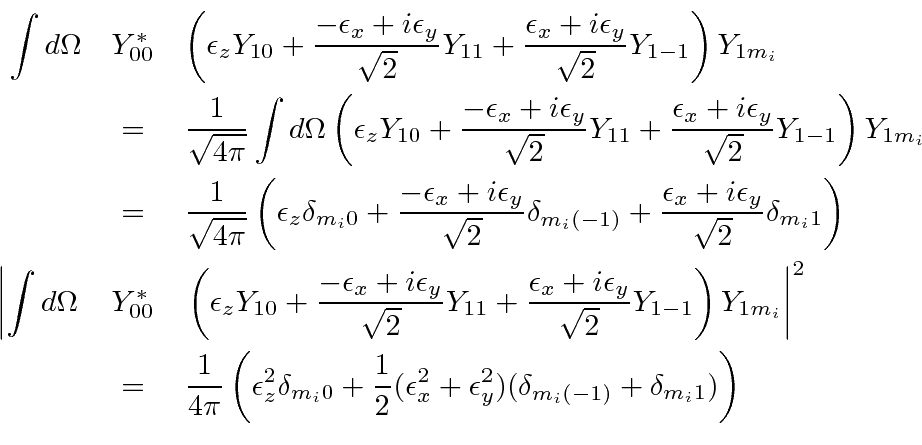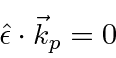## Explicit 2p to 1s Decay Rate

Starting from the summary equation for electric dipole transitions, above,we specialize to the 2p to 1s decay,perform the radial integration,and perform the angular integration.Lets assume the initial state is unpolarized, so we will sum overand divide by 3, the number of differentallowed.Our result is independent of photon polarization since we assumed the initial state was unpolarized, but, we must still sum over photon polarization. Lets assume that we are not interested in measuring the photon's polarization. The polarization vector is constrained to be perpendicular to the photons directionso there are two linearly independent polarizations to sum over. This just introduces a factor of two as we sum over final polarization states.

The integral over photon direction clearly just gives a factor ofsince there is no direction dependence left in the integrand (due to our assumption of an unpolarized initial state).Jim Branson 2013-04-22